# Measurement

Base quantities and their units; mass (kg), length (m), time (s), current (A), temperature (K), amount of substance (mol):

Base Quantities SI Units
Name Symbol
Length metre m
Mass kilogram kg
Time second s
Amount of substance mole mol
Temperature Kelvin K
Current ampere A
Luminous intensity candela cd

Derived units as products or quotients of the base units:

Derived Quantities Equation Derived Units
Area (A) A = L2 m2
Volume (V) V = L3 m3
Density (ρ) ρ = m / V kg m-3
Velocity (v) v = L / t ms-1
Acceleration (a) a = Δv / t ms-1 / s = ms-2
Momentum (p) p = m x v (kg)(ms-1) = kg m s-1

Derived Quantities Equation Derived Unit Derived Units
Special Name Symbol
Force (F) F = Δp / t Newton N [(kg m s-1) / s = kg m s-2
Pressure (p) p = F / A Pascal Pa (kg m s-2) / m2 = kg m-1 s-2
Energy (E) E = F x d joule J (kg m s-2)(m) = kg m2 s-2
Power (P) P = E / t watt W (kg m2 s-2) / s = kg m2 s-3
Frequency (f) f = 1 / t hertz Hz 1 / s = s-1
Charge (Q) Q = I x t coulomb C A s
Potential Difference (V) V = E / Q volt V (kg m2 s-2) / A s = kg m2 s-3 A-1
Resistance (R) R = V / I ohm Ω (kg m2 s-3 A-1) / A = kg m2 s-3 A-2

Prefixes and their symbols to indicate decimal sub-multiples or multiples of both base and derived units:

Multiplying Factor Prefix Symbol
10-12 pico p
10-9 nano n
10-6 micro μ
10-3 milli m
10-2 centi c
10-1 decid d

103

kilo k
106 mega M
109 giga G
1012 tera T

#### Estimates of physical quantities:

When making an estimate, it is only reasonable to give the figure to 1 or at most 2 significant figures since an estimate is not very precise.

Physical Quantity Reasonable Estimate
Mass of 3 cans (330 ml) of Coke 1 kg
Mass of a medium-sized car 1000 kg
Length of a football field 100 m
Reaction time of a young man 0.2 s
• Occasionally, students are asked to estimate the area under a graph. The usual method of counting squares within the enclosed area is used. (eg. Topic 3 (Dynamics), N94P2Q1c)
• Often, when making an estimate, a formula and a simple calculation may be involved.
##### EXAMPLE 1:

Estimate the average running speed of a typical 17-year-old‟s 2.4-km run.

velocity = distance / time = 2400 / (12.5 x 60) = 3.2 ≈3 ms-1

##### EXAMPLE 2:

Which estimate is realistic?

Option Explanation
A The kinetic energy of a bus travelling on an expressway is 30000J A bus of mass m travelling on an expressway will travel between 50 to 80 kmh-1, which is 13.8 to 22.2 ms-1. Thus, its KE will be approximately ½ m(182) = 162m. Thus, for its KE to be 30000J: 162m = 30000. Thus, m = 185kg, which is an absurd weight for a bus; ie. This is not a realistic estimate.
B The power of a domestic light is 300W. A single light bulb in the house usually runs at about 20W to 60W. Thus, a domestic light is unlikely to run at more than 200W; this estimate is rather high.
C The temperature of a hot oven is 300 K. 300K = 27 0C. Not very hot.
D The volume of air in a car tyre is 0.03 m3.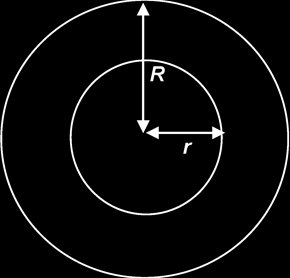Estimating the width of a tyre, t, is 15 cm or 0.15 m, and estimating R to be 40 cm and r to be 30 cm,

volume of air in a car tyre is
= π(R2r2)t
= π(0.42 – 0.32)(0.15)
= 0.033 m3
≈ 0.03 m3 (to one sig. fig.)

#### Distinction between systematic errors (including zero errors) and random errors and between precision and accuracy:

Random error: is the type of error which causes readings to scatter about the true value.

Systematic error: is the type of error which causes readings to deviate in one direction from the true value.

Precision: refers to the degree of agreement (scatter, spread) of repeated measurements of the same quantity. {NB: regardless of whether or not they are correct.}

Accuracy: refers to the degree of agreement between the result of a measurement and the true value of the quantity.

 → → R Error Higher → → → → → → Less Precise → → → ↓ ↓ ↓ S Error Higher Less Accurate ↓ ↓ ↓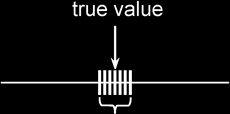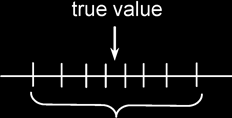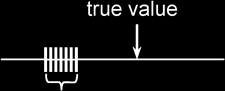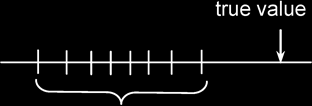Assess the uncertainty in a derived quantity by simple addition of actual, fractional or percentage uncertainties (a rigorous statistical treatment is not required).

For a quantity x = (2.0 ± 0.1) mm,

Actual/ Absolute uncertainty, Δ x = ± 0.1 mm

Fractional uncertainty, Δxx = 0.05

Percentage uncertainty, Δxx  100% = 5 %

If p = (2x + y) / 3 or p = (2x - y) / 3 , Δp = (2Δx + Δy) / 3

If r = 2xy3 or r = 2x / y3 , Δr / r = Δx / x + 3Δy / y

Actual error must be recorded to only 1 significant figure, &
The number of decimal places a calculated quantity should have is determined by its actual error.

For eg, suppose g has been initially calculated to be 9.80645 ms-2 & Δg has been initially calculated to be 0.04848 ms-2. The final value of Δg must be recorded as 0.05 ms-2 {1 sf }, and the appropriate recording of g is (9.81 ± 0.05) ms-2.

#### Distinction between scalar and vector quantities:

Scalar Vector
Definition A scalar quantity has a magnitude only. It is completely described by a certain number and a unit. A vector quantity has both magnitude and direction. It can be described by an arrow whose length represents the magnitude of the vector and the arrow-head represents the direction of the vector.
Examples Distance, speed, mass, time, temperature, work done, kinetic energy, pressure, power, electric charge etc.
Common Error:
Students tend to associate kinetic energy and pressure with vectors because of the vector components involved. However, such considerations have no bearings on whether the quantity is a vector or scalar.
Displacement, velocity, moments (or torque), momentum, force, electric field etc.

#### Representation of vector as two perpendicular components:

In the diagram below, XY represents a flat kite of weight 4.0 N. At a certain instant, XY is inclined at 30° to the horizontal and the wind exerts a steady force of 6.0 N at right angles to XY so that the kite flies freely.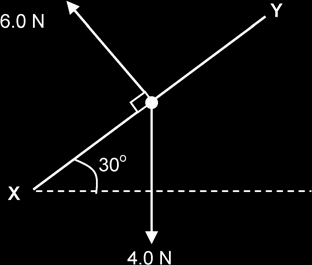By accurate scale drawing By calculations using sine and cosine rules, or Pythagoras‟ theorem

Draw a scale diagram to find the magnitude and direction of the resultant force acting on the kite.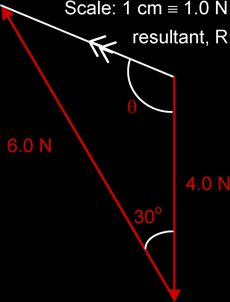R = 3.2 N (≡ 3.2 cm)
at θ = 112° to the 4 N vector.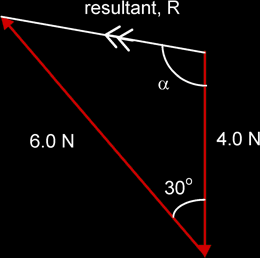Using cosine rule, a2 = b2 + c2 – 2bc cos A
R2 = 42 + 62 -2(4)(6)(cos 30°)
R = 3.23 N

Using sine rule: a / sin A = b / sin B
6 / sin α = 3.23 / sin 30°
α = 68° or 112°
= 112° to the 4 N vector

Summing Vector Components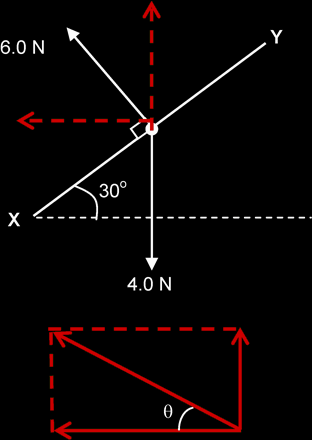Fx = - 6 sin 30° = - 3 N

Fy = 6 cos 30° - 4 = 1.2 N

R = √(-32 + 1.22) = 3.23 N

tan θ = 1.2 / 3 = 22°

R is at an angle 112° to the 4 N vector. (90° + 22°)